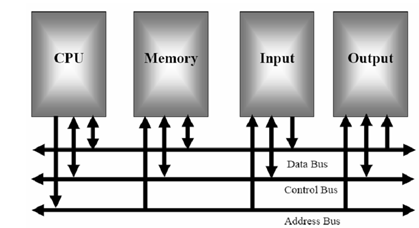## Block Diagram of Computer Assignment Help

Assignment Help: >> Introduction to 8085 Microprocessors - Block Diagram of Computer

Block Diagram of Computer:

Figure illustrates a block diagram for Von Neumann computer model. The main parts of this model are the central processing unit (CPU), memory, and output and Input circuitry or l/O. connecting these parts is three sets of parallel lines called buses. The three buses are such as data bus, address bus, and the control bus. Let's take a short view at each of these parts.Figure: Block Diagram of a Computer

The memory section generally consists of a mixture of RAM (Random Access Memory) and ROM (Read Only Memory). It can also have magnetic hard disks, magnetic floppy disks or optical disks (CDs, DVDs). Memory contains two purposes.

The first purpose is to hold the binary codes for the sequences of instructions you desire the computer to carry out. While you write a computer program, what you are in fact doing is writing a sequential list of instructions for the computer. The second purpose of the memory is to hold the binary-coded data with which the computer is going to be working. For instance, this data might be the inventory records of a supermarket.

 Address Bus Central Processing Unit Control Bus Data Bus Input and output